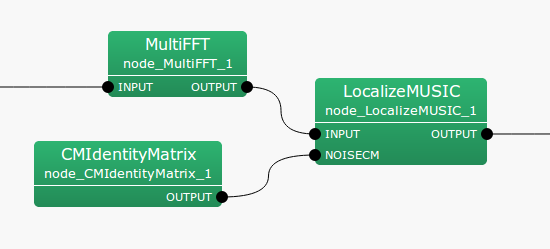## 6.2.11 CMIdentityMatrix

### 6.2.11.1 Module Overview

Outputs a correlation matrix containing a unit matrix.

None.

### 6.2.11.3 Usage

In what case is the node used?

Connect this node to the NOISECM input terminal of the LocalizeMUSIC  node to tuen OFF the noise suppression function of LocalizeMUSIC .

Typical Examples

Figure. 6.27 shows a usage example of CMIdentityMatrix node.

Since this node generates correlation matrix data with a unit matrix for all frequency bins, the input terminal does not exist. It outputs the generated correlation matrix from the output terminal.Figure 6.27: Network Example using CMIdentityMatrix

### 6.2.11.4 I/O and property setting of the node

Table 6.28: Parameter list of CMIdentityMatrix
 Parameter Type Default Unit Description NB_CHANNELS 8 Number of channels $M$ of input signal LENGTH 512 Frame length $NFFT$

Input

None.

Output

OUTPUT

: Matrix<complex<float> >  type. Correlation matrix for each frequency bin. the $M$-th order unit matrix correlation matrix outputs $NFFT/2 + 1$ items. Matrix<complex<float> >  contains rows corresponding to the row of frequencies ($NFFT/2 + 1$ rows), and columns containing the complex correlation matrix ($M * M$ columns across).

Parameter

NB_CHANNELS

: int  type. Number of channels for input signal. Equivalent to the order of the correlation matrix. Must be matched with the order of the former correlation matrix used. Default value is 8.

LENGTH

: int  type. Default value is 512. FFT points at the time of Fourier transform. Must be matched till the former FFT length values.

### 6.2.11.5 Module Description

For an $M$-th order complex square array with a correlation matrix for each frequency bin, it stores the unit matrix, and corrects and outputs the result in Matrix<complex<float> >  format.